### Description

This paper proposes an end-to-end deep encoder-decoder network for 3D biomedical segmentation. The network is a combination of three main parts: multi-modal encoder, cross-modality convolution, and convolutional LSTM.

Four different modalities of (MRI) image are commonly referenced for the brain tumor surgery: T1, T1C, T2, and FLAIR. As shown below, the slices from four different modalities are stacked together along the axial orientation. Then, they pass through different CNNs in the multi-modal encoder (each CNN is applied to a different modality) to obtain a semantic latent feature representation. Latent features from multiple modalities are effectively aggregated by the proposed cross-modality convolution layer. Then, convolutional LSTM exploits the spatial and sequential correlations of consecutive slices. Finally, a 3D image segmentation is generated by concatenating a sequence of 2D prediction results. This model tries to jointly exploit the correlations between different modalities and the spatial and sequential dependencies for consecutive slices.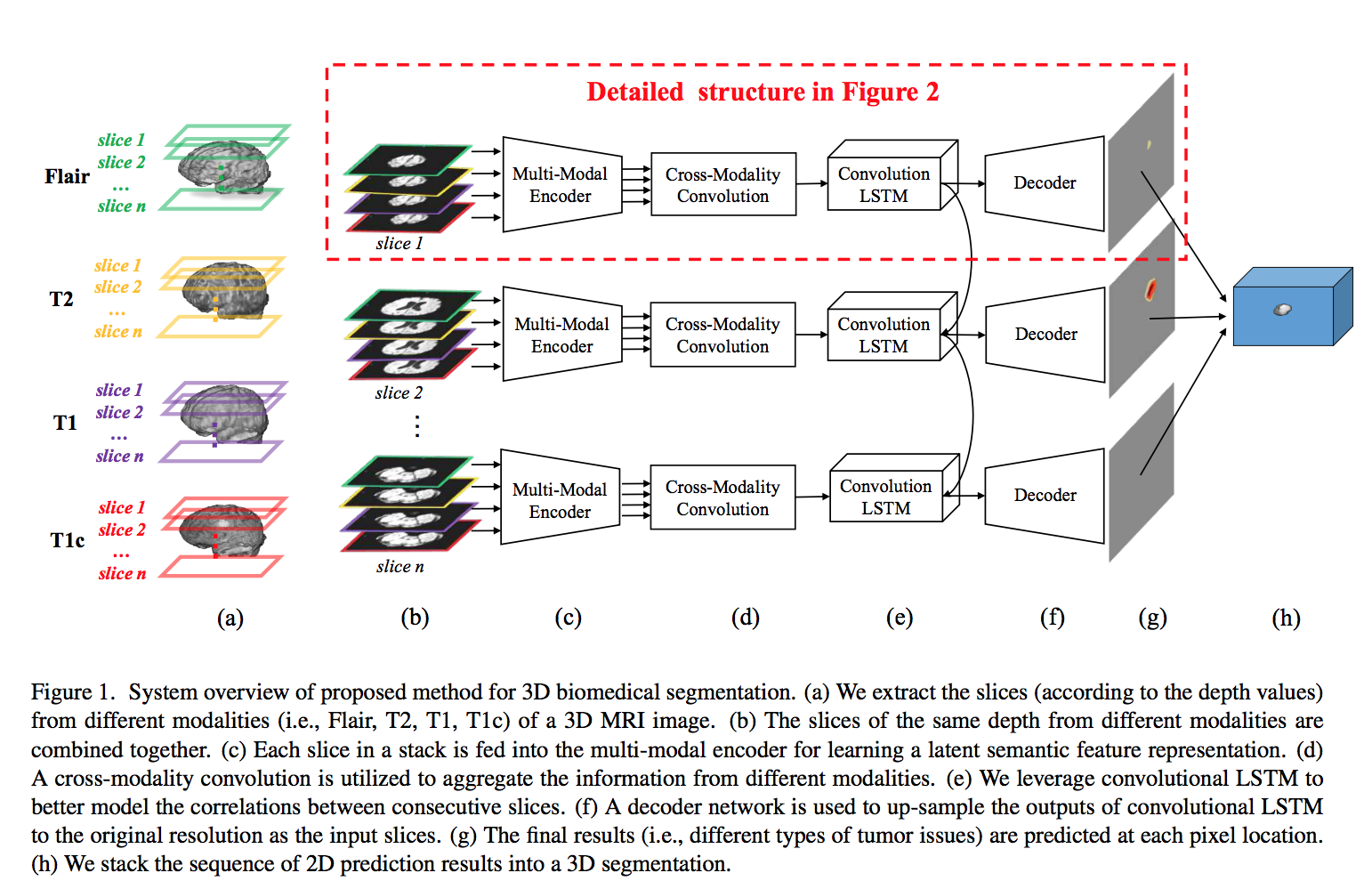The encoder is used for extracting the deep representation of each modality. As shown below, four different slices with the same depth fed into the encoder with four convolution layers and four max-pooling layers. Each modality is encoded to a feature map of size h × w × C (h, w are feature dimensions, C is number of channels ). Then, the features of the same channels from four modalities are stacked into one stack. The cross-modality convolution(CMC) performs 3D convolution with the kernel size 4 × 1 × 1, where 4 is the number of modalities. The output as a sequence of slices fed into convLSTM to model the slice dependencies. Decoder up-samples the feature maps to the original resolution for predicting the dense results. Then, pass the output of the decoder to a multi-class soft-max classifier to produce the class probabilities of each pixel.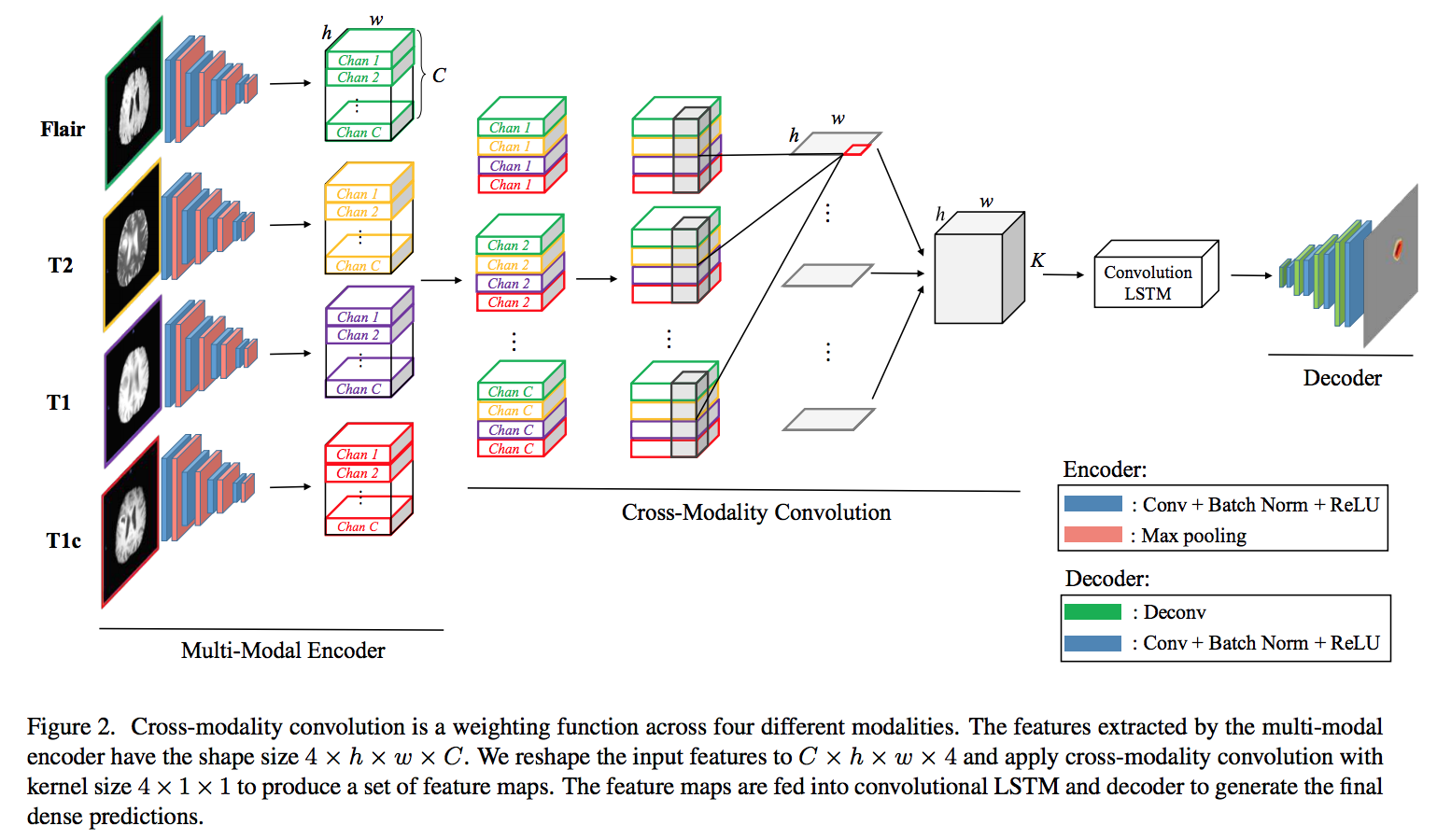Feature concatenation often requires additional learnable weights because of the increase of channel size. The authors use multi-resolution feature maps(multiplication Resolution Feature or MRF) instead of concatenation. They perform CMC after each pooling layer in the multi-modal encoder and multiply it with the up-sampled feature maps from the decoder to combine multi-resolution and multi-modality information.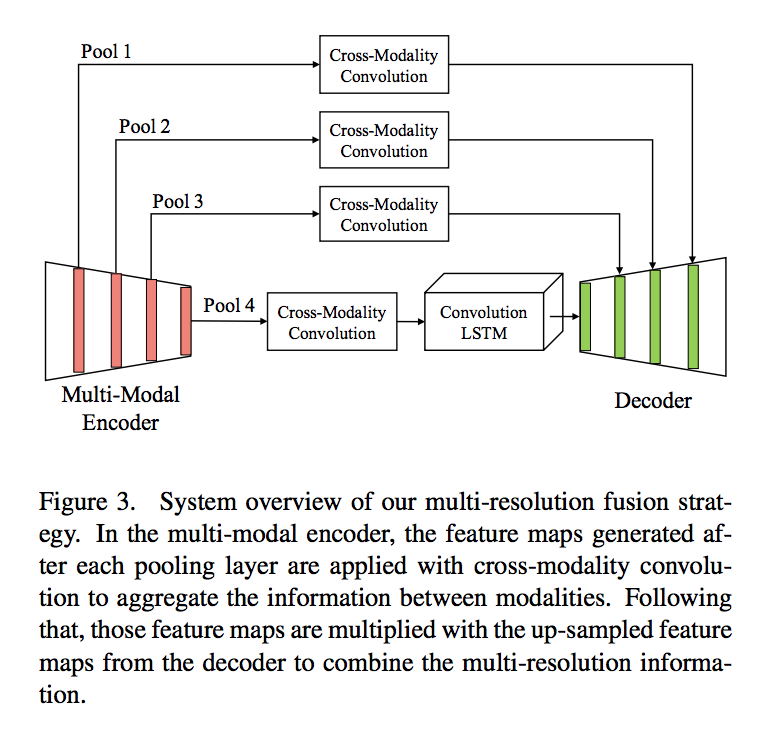The label image contains five labels: non-tumor, necrosis, edema, non-enhancing tumor and enhancing tumor. The evaluation system separates the tumor structure into three regions due to practical clinical applications:

1. Complete score: it considers all tumor areas and evaluates all labels 1, 2, 3, 4 (0 for normal tissue, 1 for edema, 2 for non-enhancing core, 3 for necrotic core, and 4 for enhancing core).

2. Core score: it only takes tumor core region into account and measures the labels 1, 3, 4.

3. Enhancing score: it represents the active tumor region, only containing the enhancing core (label 4) structures for high-grade cases.

There are three kinds of evaluation criteria: Dice, Positive Predicted Value and Sensitivity.Where T1 is the true lesion area and P1 is the subset of voxels predicted as positives for the tumor region.

$Dice = \frac{P_{1} \cap T_{1}}{(P_{1} + T_{1} )/2}$ $PPV = \frac{P_{1} \cap T_{1}}{P_{1}}$ $Sensivity = \frac{P_1 \cap T_{1}}{T_{1}}$

### Results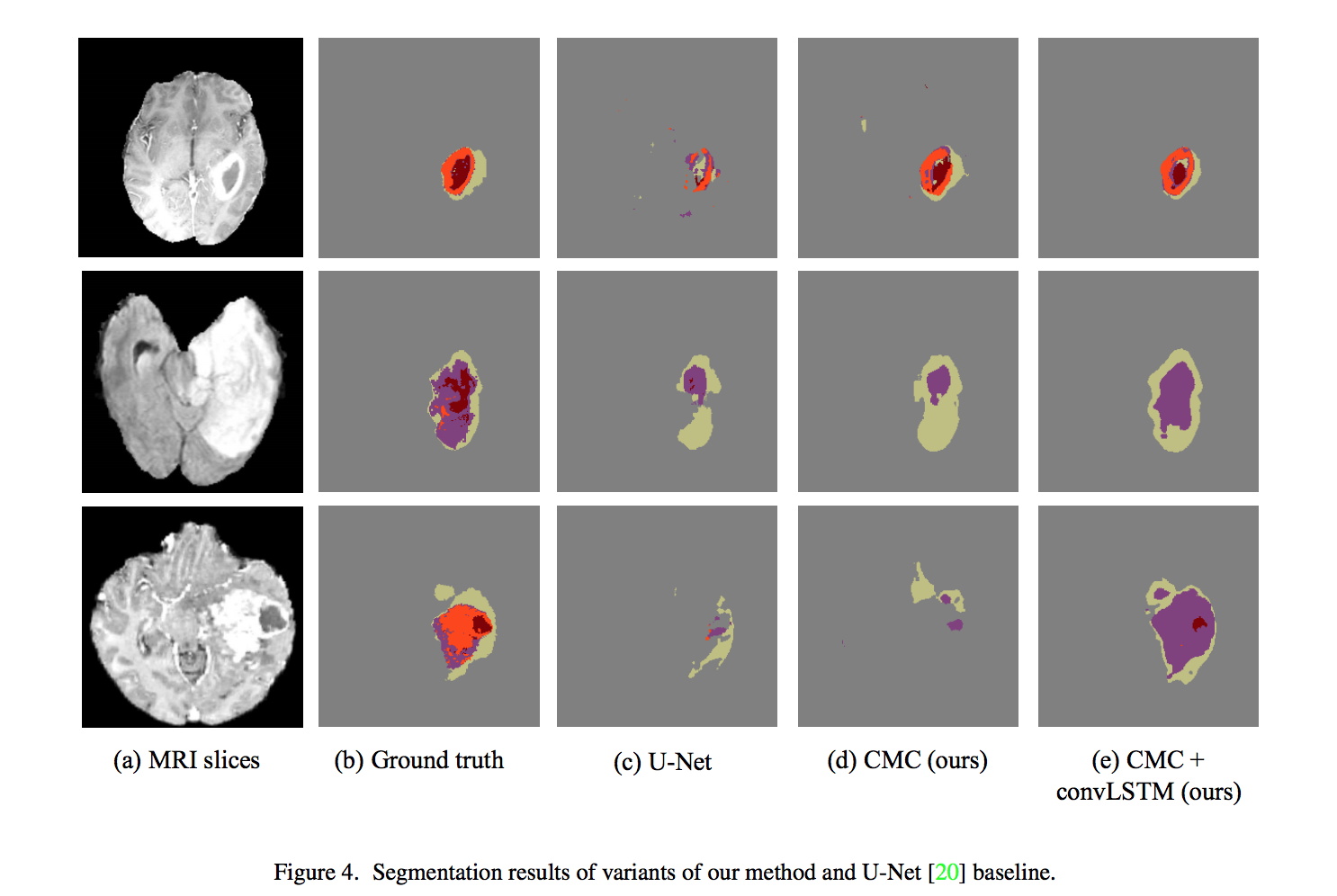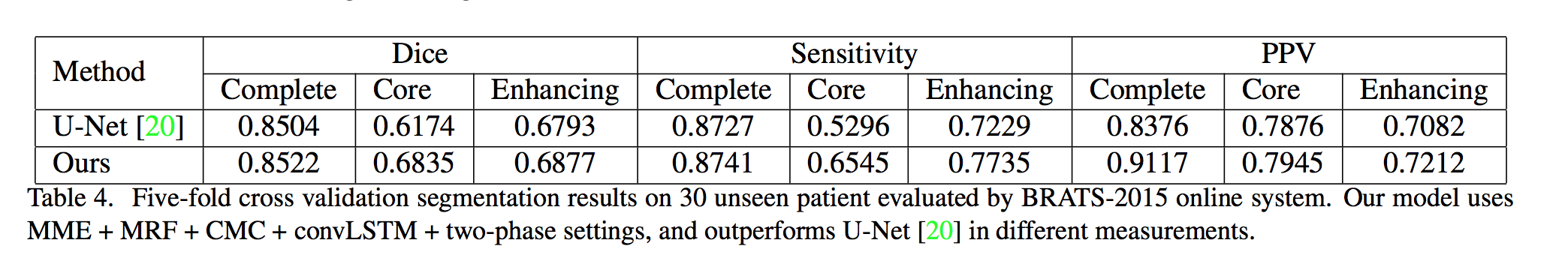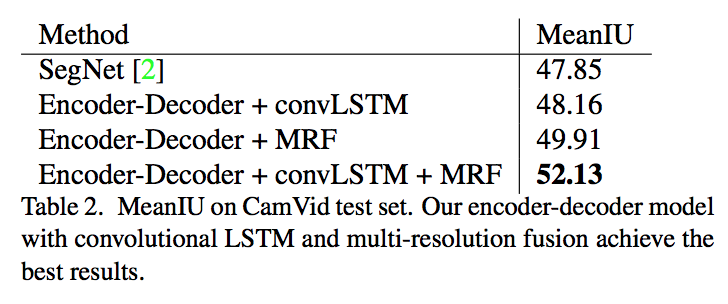Note: This work is most related to KU-Net, and different from it, they propose a CMC to better combine the information from MRI data, and jointly optimize the slice sequence learning and CMC in an end to end manner.

Paper of KU-Net can be found here